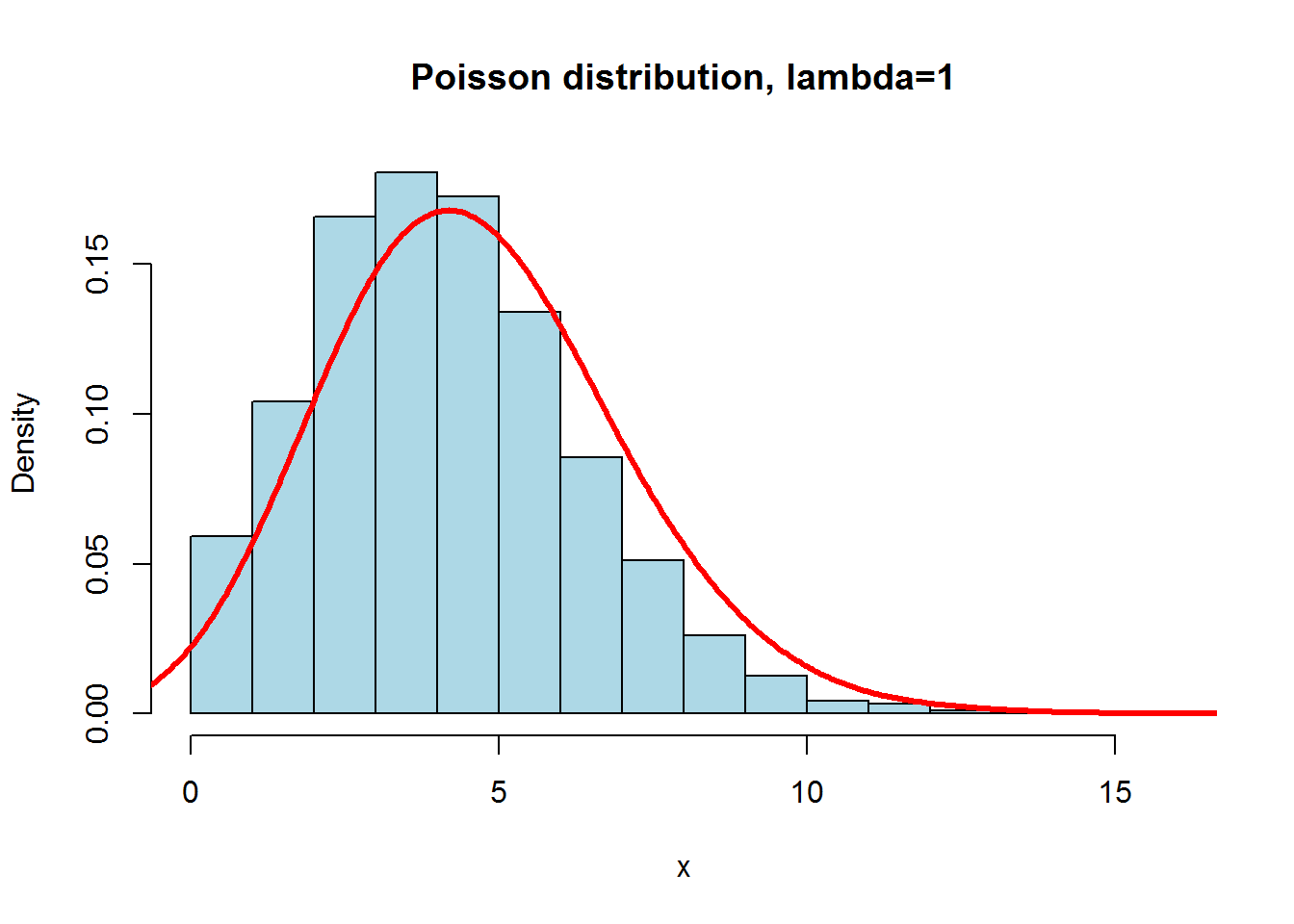### General description

The Poisson distribution is a discrete probability distribution

• A discrete probability distribution models variables who’s values are countable
• I.e. Number of e-mails received in a day
• Note that discrete variables can only be whole numbers (as receiving 5.5 emails in a day does not make sense)
• This contrasts with a continuous probability distribution like the normal distribution

The Poisson distribution models the probability of obtaining particular count of a discrete variable, given the average count of that variable

• This model is represented as $$f(x) = \frac{\lambda^xe^{-\lambda}}{x!}$$
• $$f(x) =$$ probability of discrete variable at value $$x$$
• $$\lambda =$$ mean of the discrete variable being modeled
• For the Poisson distribution, the variance ($$\sigma^2$$) is equal to the mean ($$\lambda$$ or $$\mu$$)

### Examples

• For Pu-239, with an average of 2.3 decays per second, what is the probability of observing 3 decays over a period of two seconds?
• $$\lambda = (2.3 decays/second)*(2 seconds) = 4.6 seconds$$
• $$x = 3$$
• $$f(x) = \frac{4.6^3e^{-4.6}}{3!} = 0.163$$
• With R:

dpois(x = 3, lambda = 4.6) #Probability of observing EXACTLY 3 events
##  0.1630676
ppois(q = 3, lambda = 4.6) #Probability of observing 3 events OR LESS
##  0.3257063
ppois(q = 3, lambda = 4.6, lower.tail = FALSE) #Probability of observing MORE THAN 3 events
##  0.6742937
• Plotting the distribution in R

N <- 10000 #Arbitrarily large number of values R should generate from modeled distribution
x <- rpois(N, lambda = 4.6) #Generates N-number values from indicated poisson distribution
hist(x,
xlim=c(min(x),max(x)), probability=T, nclass=max(x)-min(x)+1,
col='lightblue',
main='Poisson distribution, lambda=1')
lines(density(x,bw=1), col='red', lwd=3)### Poisson vs. binomial distribution

The binomial distribution

• The binomial distribution models the probaility of observing a certain frequency of events given the average frequency of the event and the number of events observed
• Represented as $$f(x) = ( \begin{array}{r}n \\ k \end{array} ) p^k(1-p)^{(n-k)}$$
• $$n =$$ number of total observations (“trials”)
• $$k =$$ number of outcomes of interest (“successes”)
• $$( \begin{array}{r}n \\ k \end{array} ) = n$$ choose $$k =$$ the number of ways you can draw k-numbered outcomes out of n-values
• I.e $$( \begin{array}{r}2 \\ 3 \end{array} ) = 3$$ since $$n = \{1,2,3\}$$ and outcomes with $$k=2$$ are $$\{1,2\},\{1,3\},\{2,3\}$$ (3 outcomes)
• Calculated as $$( \begin{array}{r}n \\ k \end{array} ) = \frac{n!}{k!(n-k)!}$$ or in R as choose(n,k)
• $$p =$$ probability of the outcome of interest (“probability of a success”)
• Example - “What is the probability of obtaining a p-value of 0.05 in 20 comparisons of random data”
• A p-value of 0.05 is equivalent to 1 “success” in 20 so:
• $$n = 20, k = 1, p = 0.05$$
dbinom(x=1,size=20, prob=0.05) #Probability of obtaining 1 positive p-value from 20 random comparisons
##  0.3773536
pbinom(q=1-1,size=20, prob=0.05, lower.tail = FALSE) #Probability of obtaining AT LEAST 1 positive p-value from 20 random comparisons ("-1", since lower.tail = F isn't inclusive)
##  0.6415141